×

Search anything:

# Word Embeddings: GloVe and Word2Vec

#### Machine Learning (ML)Get this book -> Problems on Array: For Interviews and Competitive Programming

Word Embeddings correlates the likeness of the meaning of words with their relative similarity and represent them numerically as a vector.

In this article, we are going to see What is Word Embeddings? And why we need it? First of all, come to our second question's answer: We need it because

• It is obvious that while working with an algorithm or mathematical system we need some type of numeric input. Since words initially in the corpus are not in numeric format. Hence, it solves this problem by representing the words numerically, usually in the shape of a vector of dimension d.
• How do we make a computer understand that “Charger” in “I want my charger to charge a mobile” is a charger that can charge mobile and not a laptop? The solution to this problem lies in making representation for words that catch their implications, semantic connections and the various sorts of context they are utilized in.

In simplified terms, Word Embedding is the representation of the word in numeric format, usually in the shape of a vector of dimension d. And there may be various numerical representations for the same word.

In formal terms, Word Embedding is a procedure that treats words as vectors whose relative likenesses associate with semantic closeness. Earlier, Natural language processing (NLP)systems generally encode words as strings, which are subjective and give no valuable data to the system concerning the connections that may exist between various words. But now this problem is solved by applying the word embedding technique to gain insight into words.

To have a clear view take an example, let’s take the words India, Delhi, America, and Washington. We can use basic arithmetical operations to discover semantic similarities. Estimating likeness between vectors is computable using measures, such as cosine similarity. So when we subtract the vector of the word Delhi from the vector of the word India, then its cosine distance would be close to the distance between the word Washington minus the word America.

`W("India")−W("Delhi") ≃ W("America")−W("Washington")`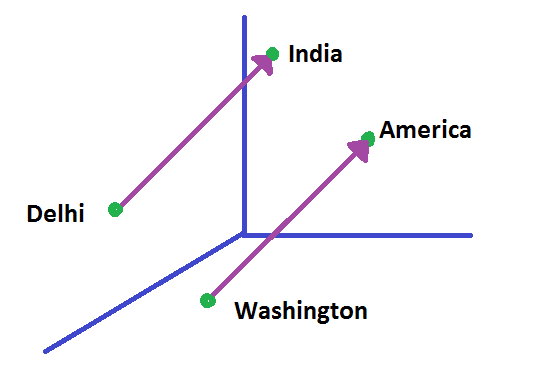Word embeddings Methods can be divided into two main categories:

• Count / Frequency-based Methods: In this method, the meaning of a word is defined "by the company it keeps" (also known as Firth’s hypothesis). This hypothesis leads to a very simple though a very high-dimensional word embedding. This approach is unsupervised. The models created by this method fully depends on the co-occurrence matrix and word frequency.
• Context / Prediction-based Methods: In this method, it builds a predictive model that predicts a word from its neighbors in terms of learned small, dense embedding vectors. This approach is supervised. In this method, the model learns the word embedding representation efficiently.

## GloVe (Global Vectors)

The GloVe model is one of the unsupervised learning algorithm log-bilinear model for learning word embedding or obtaining numerical(vector) representation of words, developed by Stanford. The core insight of the GloVe Model is the simple observation that the likelihood of word-word co-occurrence ratios has the capacity to represent some type of significance. GloVe works by using the global matrix factorization.

May be sometimes a question struck in your mind that Why for all Unsupervised Methods we use word occurrences statistics in a corpus as a primary source for learning word representations? Lets take a simple example to get the clear view that what's going on exactly:

• We know that Water has two thermodynamic phases ice(~0 Degree Celsius) and steam(~100 Degree Celsius).
• Take i = ice and j = steam. We now examine the relationship of these words by taking the ratio of co-occurrence probabilities with other possibe words related to them, let say k.
• For k = solid, which is related to ice rather than steam the Pik/Pjk ratio will be large.
• For k = gas, which is related to steam rather than gas the Pik/Pjk ratio will be small.
• For k = water/fashion i.e., they either related to both ice and steam or to neither the Pik/Pjk ratio will be around one.
• In below table as mentioned it shows the probabilities and their ratios and the numbers which confirms the above scenario: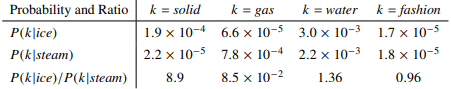The ratio Pik/Pjk is better able to distinguish between relevant(solid, gas) and irrelevant(water,fashion) words and also able to distinguish between relevant words so accurately.

## Word2Vec

The word2vec is the most popular and efficient predictive model for learning word embeddings representations from the corpus, created by Mikolov et al. in 2013. It comes in two flavors, the Continuous Bag-of-Words model(CBOW) and the Skip-Gram model. At a much lower computational cost, the Word2Vec model showed a huge improvement in efficiency and accuracy. Algorithmically, the CBOW and the Skip-Gram model are similar but there is a difference in these models, the Skip-Gram model by taking the target words predicts the source context words. While the Continuous Bag-of-Words model does opposite, by taking the source context words it predicts the target words. Here target words are the center words while the contexts are surrounding words. For Example in the sentence "Today's Weather is too hot". In this, we take [Weather] as a target word and [Today's, is, too, hot] as the context words. For having a clear view you can see the below example: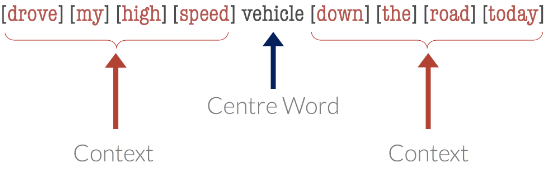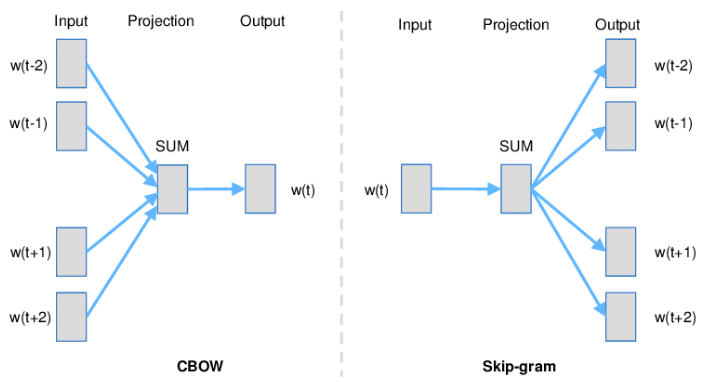## Implementation

Here we implement the word2vec algorithm on “Game of Thrones” data. And for this we use the gensim module that implements the word2vec family of algorithms directly without any complexity:

## To Extract Words

``````import sys
from nltk.corpus import stopwords
from nltk.tokenize import sent_tokenize

STOP_WORDS = set(stopwords.words('english'))

def get_words(txt):
return filter(
lambda x: x not in STOP_WORDS,
re.findall(r'\b(\w+)\b', txt)
)

def parse_sentence_words(input_file_names):
"""Returns a list of a list of words. Each sublist is a sentence."""
sentence_words = []
for file_name in input_file_names:
for line in open(file_name):
line = line.strip().lower()
line = line.decode('unicode_escape').encode('ascii','ignore')
sent_words = map(get_words, sent_tokenize(line))
sent_words = filter(lambda sw: len(sw) > 1, sent_words)
if len(sent_words) > 1:
sentence_words += sent_words
return sentence_words

# You would see five .txt files after unzip 'a_song_of_ice_and_fire.zip'
input_file_names = ["001ssb.txt", "002ssb.txt", "003ssb.txt",
"004ssb.txt", "005ssb.txt"]
GOT_SENTENCE_WORDS= parse_sentence_words(input_file_names)

#To provide a word2vec model
from gensim.models import Word2Vec
# size: the dimensionality of the embedding vectors.
# window: the maximum distance between the current and predicted word within a sentence.
model = Word2Vec(GOT_SENTENCE_WORDS, size=128, window=3, min_count=5, workers=4)
model.wv.save_word2vec_format("got_word2vec.txt", binary=False)
``````

It gives the result:

To get the top ten similar words to "king" in Game of thrones Corpus Word embedding space:

``````model.most_similar('king',topn=10)
``````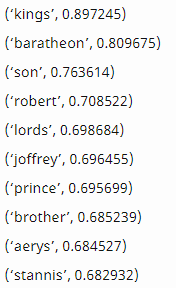To get the top ten similar words to "queen" in Game of thrones Corpus Word embedding space:

``````model.most_similar('queen',topn=10)
``````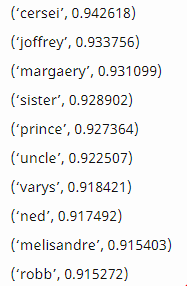## Applications

You must already have come across some examples of why word embeddings are used. Some interesting, and more specific examples are:

• Word Embedding is used in Sentiment Analysis.
• Used in Analyzing Survey Responses.
• Used in the Music/Books/Video Recommendation System.#### Nikunj Bansal

Data Scientist@Cognizant | MLOps | Researcher

Word Embeddings: GloVe and Word2Vec